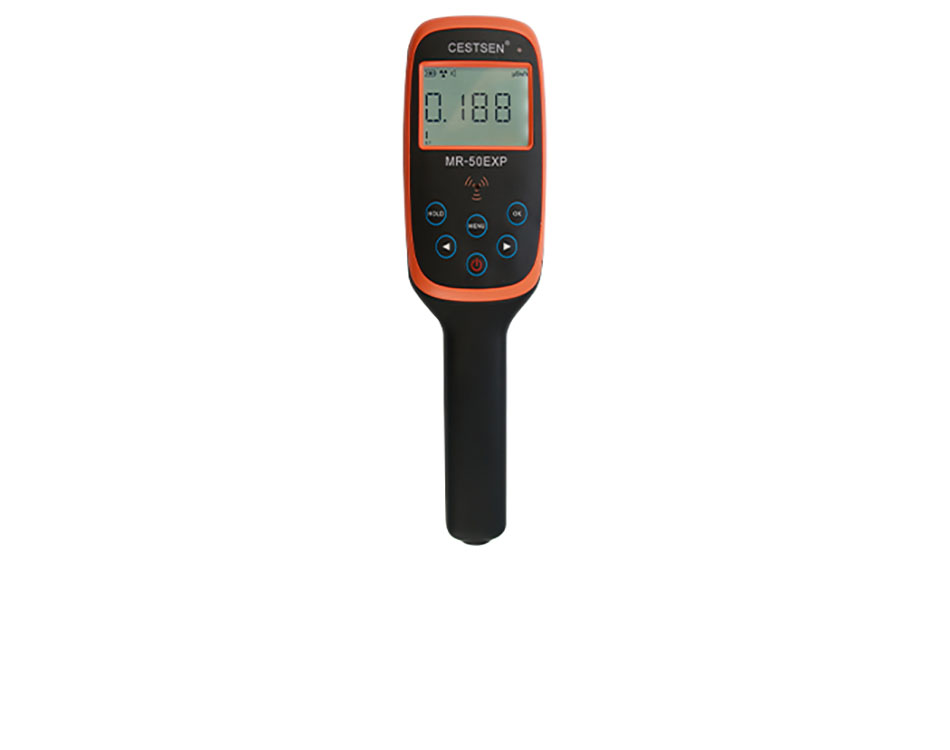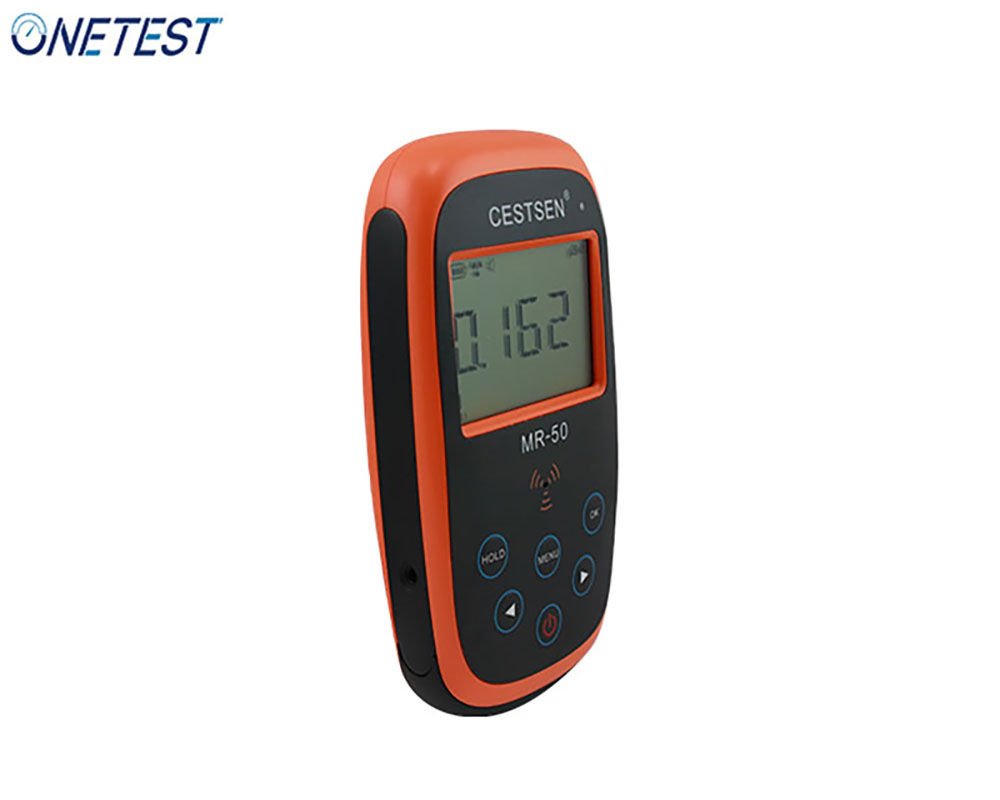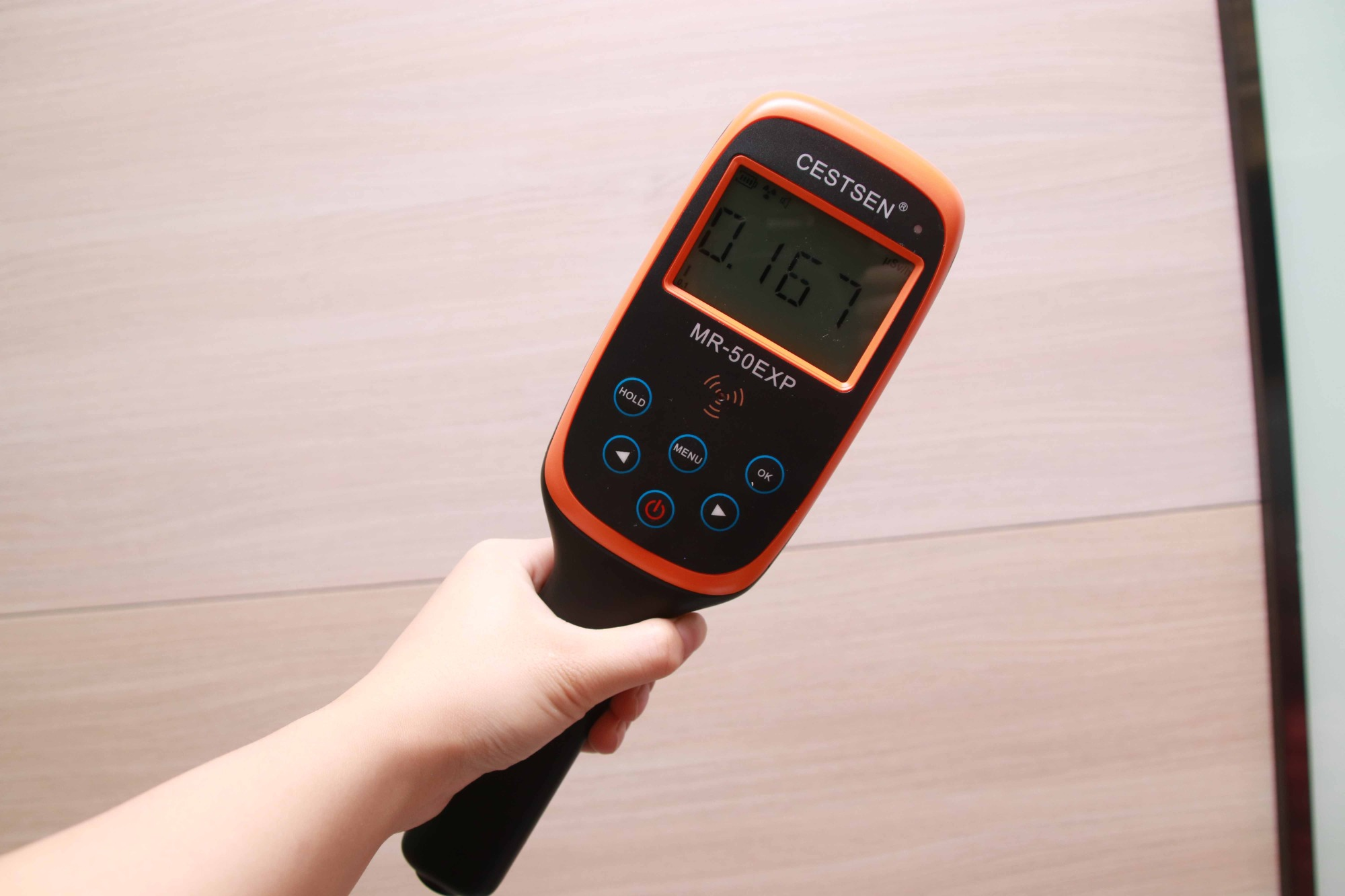News Category

# How to understand the quantity and unit of radiation dose

Views : 533
Update time : 2022-10-28 16:51:48
Users who have used the radiation dosimeter should be familiar with the radiation quantity and unit, but it will be difficult to actually interpret them. So today we will introduce how to understand the radiation quantity and unit.It refers to the number of atoms decayed per second of radioactive elements or isotopes, and its unit is Becquerel, or Bq for short. Becquerel is named mainly to commemorate the French scientist who first discovered natural radioactive substances more than 100 years ago. 1Bq is defined as one atomic nucleus decaying every second.

absorbed dose

Absorbed dose refers to the most basic dosimetric physical quantity, usually refers to the radiation measurement absorbed by a unit mass object in the process of interaction between rays and objects. The unit is Gray, Gy, 1Gy=1 J/kg. The special noun is Gray, the symbol "Gy", 1Gy=1 J · kg-1. This is a large unit, so in practical application, it is often used as the unit of one thousandth or one millionth of it, namely mGy μ Gy, even smaller, nGy.

The absorbed dose can be applied to any type of radiation and irradiated material. When we are carrying out γ In radiation monitoring, nGy/h is usually used as the measurement unit (absorbed dose rate unit), which means the hourly absorbed dose value of the measurement site. The normal natural background radiation level varies according to different regions, generally between tens and 200 nGy/h.

The absorbed dose rate of air refers to the measurement of the radiation energy absorbed by the object of unit mass in unit time, and the unit is Gy/h.Equivalent dose

Different types of radiation produce different biological effects, so even the same absorbed dose may not produce the same degree of biological effects. In order to use the same scale to express the severity or probability of biological effects caused by different types of ionizing radiation on the human body, we have adopted equivalent dose, which is a quantity taking into account the radiation weight factor.

The unit of equivalent dose is also J · kg-1. In order to distinguish it from the terminology of absorbed dose unit, the equivalent dose unit has a special name called Sievert, which is abbreviated as "Xi", and the symbol is "Sv". In practical applications, mSv μ Sv、nSv。

In the actual measurement of radiation energy, if there are multiple rays, equivalent dose units shall be used.

Effective dose

In order to describe the size of the health hazards caused by radiation and quantitatively evaluate the size of the possible risks caused by radiation exposure, the concept of effective dose was artificially introduced in the radiation protection evaluation.

In practice, the relationship between the probability of occurrence of random effects (cancer and other diseases) caused by radiation exposure and the equivalent dose is also related to the irradiated tissues or organs, because the sensitivity of various tissues and organs to radiation is different, and any exposure to the human body almost always involves more than one organ or tissue. In order to calculate the total risk of radiation to relevant organs and tissues exposed to radiation, the concept of tissue weight factor is introduced in radiation protection. The effective dose is generated after taking this factor into account. It can be said that this is a quantity that takes into account both the type of radiation and the weight factor of organs and tissues. The unit of effective dose is Sivert/Sv, named after the famous Swedish nuclear physicist Sivert. Schiavot is a unit with a large value. In practical applications, millisievert/millisievert (mSv) or microsievert/microsievert is usually used more（ μ Sv），1Sv = 1000mSv; 1mSv = 1000 μ Sv。

1 Roentgen (R)=10000 microsieverts（ μ Sv)=1 rem=1Gy=1 J · kg-1The above is the interpretation of the quantity and unit of radiation dose.